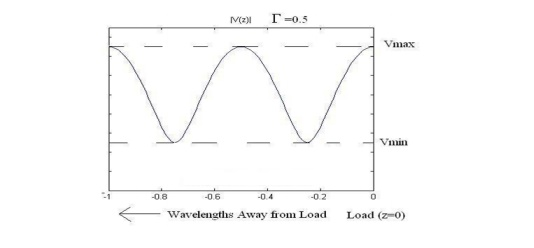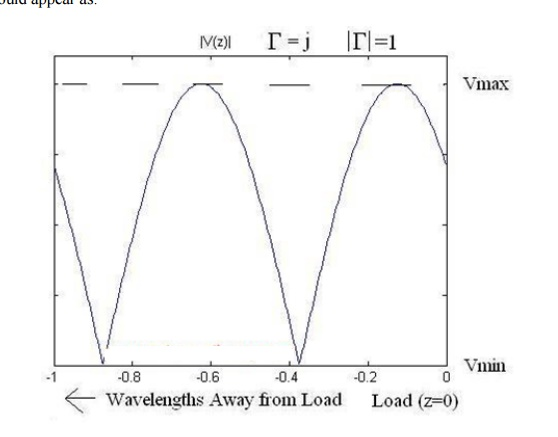Home | | Transmission Lines and Wave Guides | Measurement of VSWR and Wavelength

# Measurement of VSWR and Wavelength

Standing waves on the transmission line. Assuming the propagation constant is purely imaginary (lossless line), We can re-write the voltage and current waves as:

MEASUREMENT OF VSWR AND WAVELENGTH

## Standing Waves

Standing waves on the transmission line. Assuming the propagation constant is purely imaginary (lossless line), We can re-write the voltage and current waves as:If we plot the voltage along the transmission line, we observe a series of peaks and minimums, which repeat a full cycle every half-wavelength. If gamma equals 0.5 (purely real), then the magnitude of the voltage would appear as:Similarly, if gamma equals zero (no mismatch loss) the magnitude of the voltage would appear as:Finally, if gamma has a magnitude of 1 (this occurs, for instance, if the load is entirely reactive while the transmission line has a Z0 that is real), then the magnitude of the voltage would appear as:One thing that becomes obvious is that the ratio of Vmax to Vmin becomes larger as the reflection coefficient increases. That is, if the ratio of Vmax to Vmin is one, then there are no standing waves, and the impedance of the line is perfectly matched to the load. If the ratio of Vmax to Vmin is infinite, then the magnitude of the reflection coefficient is 1, so that all power is reflected. Hence, this ratio, known as the Voltage Standing Wave Ratio (VSWR) or standing wave ratio is a measure of how well matched a transmission line is to a load. It is defined as:The reflection coefficient may also be established using other field or circuit quantities.

The reflection coefficient can be given by the equations below, where ZS is the impedance toward the source, ZL is the impedance toward the load:Simple circuit configuration showing measurement location of reflection coefficient.Notice that a negative reflection coefficient means that the reflected wave receives a 180°, or π, phase shift.Thus the absolute magnitude (designated by vertical bars) of the reflection coefficient can be calculated from the standing wave ratio.

Study Material, Lecturing Notes, Assignment, Reference, Wiki description explanation, brief detail
Transmission Lines and Waveguides : High Frequency Transmission Lines : Measurement of VSWR and Wavelength |